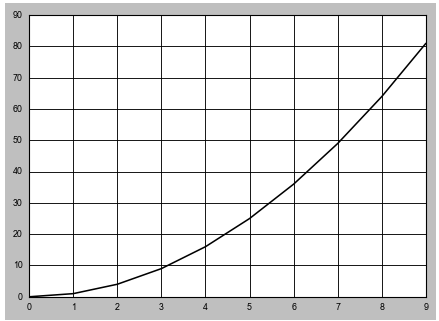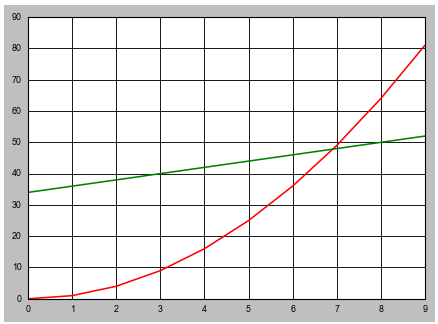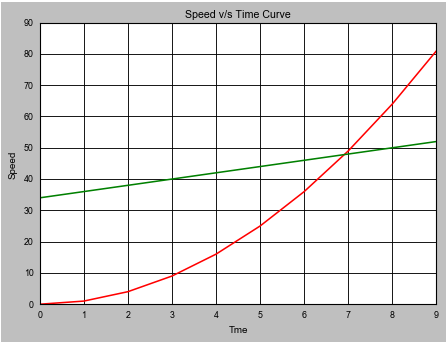# Line Plots

`x=np.arange(10)y1= x**2y2=2*x+34print(x)print(y1)print(y2)Output---------[0 1 2 3 4 5 6 7 8 9][ 0  1  4  9 16 25 36 49 64 81][34 36 38 40 42 44 46 48 50 52]`
`plt.plot(x,y1)plt.show`
`themes=plt.style.availableprint(themes)`
`plt.style.use(“seaborn-paper”)plt.plot(x,y1)plt.show`Using the seaborn paper theme we got the gray colour border and curve, and grd on the graph.
`plt.style.use(“seaborn-paper”)plt.plot(x,y1, color=”red”)plt.plot(x,y2, color=”green”)plt.show`We can plot different curves on same plot using different colors
`plt.xlabel(“Time”)plt.ylabel(“Speed”)plt.title(“Speed v/s Time Curve”)`We got the label for x and y axis along with the title for curve.
`plt.style.use(“seaborn-paper”)plt.plot(x,y1, color=”red”, label=”A”)plt.plot(x,y2, color=”green”, label=”B”)plt.legend()plt.xlabel(“Tme”)plt.ylabel(“Speed”)plt.title(“Speed v/s Time Curve”)plt.show`
`plt.plot(x,y1, color=”red”, label=”A”, linestyle=”dashed”)`
`plt.plot(x,y1, color=”red”, label=”A”, linestyle=”dashed”, marker=”o”)`

“You can achieve simplicity in the design of effective charts, graphs and tables by remembering three fundamental principles: restrain, reduce, emphasize.”

--

--